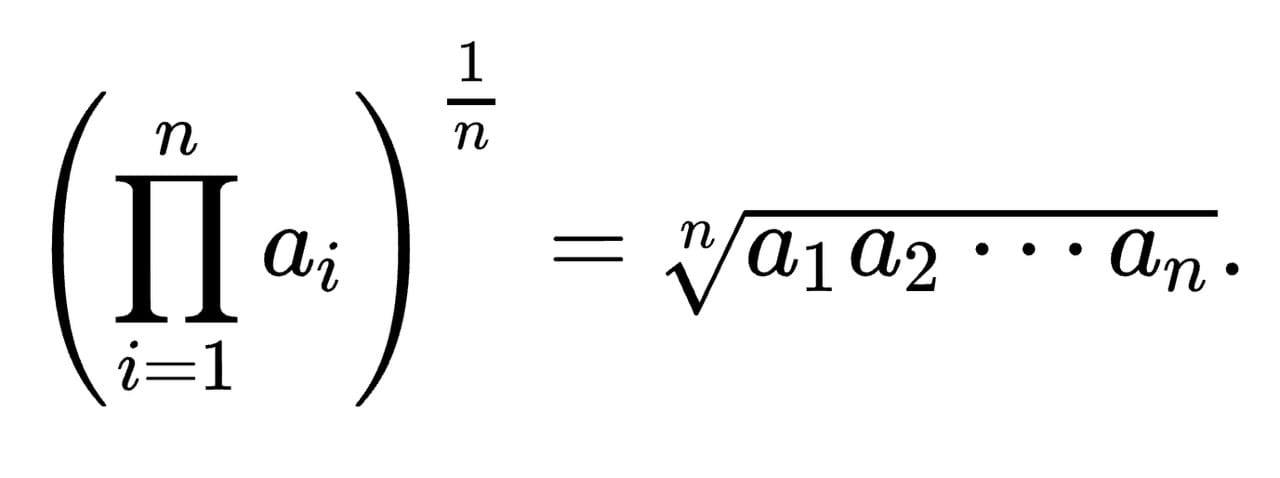﻿

# 統計：一個簡單的幾何平均數的運算範例## 幾何平均數（Geometric mean）的「單純數列」計算範例

``````1 x 4 x 5 x 6 x 7 x 3 x 8 x 4 x 9 = 725760
725760 ^ (1/9) = 4.4791（GM）
``````

## 幾何平均數（Geometric mean）的「定存年利率」計算範例

``````(1.015 ^ 2.3) X (1.021 ^ 1.2) X (1.018 ^ 0.8) X (1.0175 ^ 1.5) = 1.1046
(1.1046 ^ (1/(2.3 + 1.2 + 0.8 + 1.5))) - 1 = 1.73%（GM）
``````

## 幾何平均數（Geometric mean）的「年化報酬率」計算範例

``````第一年  10%

``````

``````//先統一將每年的報酬率加一消除負獲利，再將其相乘。
1.1 X 1.08 X 0.88 X 1.05 X 1.07 = 1.1745
1.1745 ^ (1/5) = 1.0327

//將計算出來的幾何平均值減去一，回歸正常
1.032 - 1 = 3.27%
``````